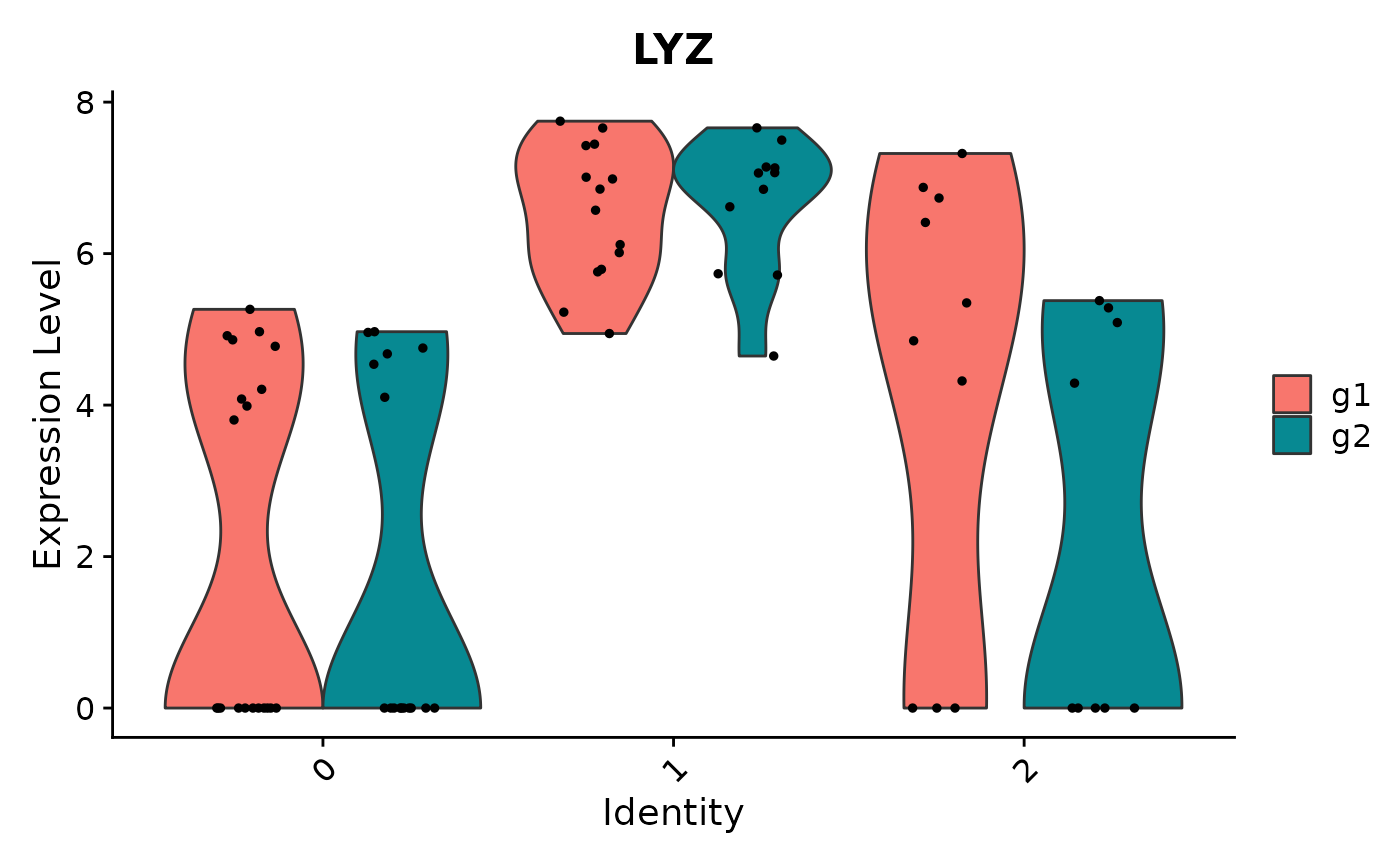Draws a violin plot of single cell data (gene expression, metrics, PC scores, etc.)

VlnPlot(
object,
features,
cols = NULL,
pt.size = NULL,
idents = NULL,
sort = FALSE,
assay = NULL,
group.by = NULL,
split.by = NULL,
y.max = NULL,
same.y.lims = FALSE,
log = FALSE,
ncol = NULL,
slot = "data",
split.plot = FALSE,
stack = FALSE,
combine = TRUE,
fill.by = "feature",
flip = FALSE,
raster = NULL
)

## Arguments

object

Seurat object

features

Features to plot (gene expression, metrics, PC scores, anything that can be retreived by FetchData)

cols

Colors to use for plotting

pt.size

Point size for geom_violin

idents

Which classes to include in the plot (default is all)

sort

Sort identity classes (on the x-axis) by the average expression of the attribute being potted, can also pass 'increasing' or 'decreasing' to change sort direction

assay

Name of assay to use, defaults to the active assay

group.by

Group (color) cells in different ways (for example, orig.ident)

split.by

A variable to split the violin plots by,

y.max

Maximum y axis value

same.y.lims

Set all the y-axis limits to the same values

log

plot the feature axis on log scale

ncol

Number of columns if multiple plots are displayed

slot

Slot to pull expression data from (e.g. "counts" or "data")

split.plot

plot each group of the split violin plots by multiple or single violin shapes.

stack

Horizontally stack plots for each feature

combine

Combine plots into a single patchworked ggplot object. If FALSE, return a list of ggplot

fill.by

Color violins/ridges based on either 'feature' or 'ident'

flip

flip plot orientation (identities on x-axis)

determine if adding a small noise for plotting

raster

Convert points to raster format. Requires 'ggrastr' to be installed.

## Value

A patchworked ggplot object if combine = TRUE; otherwise, a list of ggplot objects

FetchData

## Examples

data("pbmc_small")
VlnPlot(object = pbmc_small, features = 'PC_1')VlnPlot(object = pbmc_small, features = 'LYZ', split.by = 'groups')
#> The default behaviour of split.by has changed.
#> Separate violin plots are now plotted side-by-side.
#> To restore the old behaviour of a single split violin,
#> set split.plot = TRUE.
#>
#> This message will be shown once per session.